Courses

# Applications Of Gauss Law Class 12 Notes | EduRev

## JEE : Applications Of Gauss Law Class 12 Notes | EduRev

The document Applications Of Gauss Law Class 12 Notes | EduRev is a part of the JEE Course Physics Class 12.
All you need of JEE at this link: JEE

APPLICATIONS OF GAUSS LAW
Gauss law is one of the four fundamental equations of Electromagnetism. Gauss Law is used to evaluate Electric Field due to continuous charge systems with symmetry. Though, it is applicable everywhere, it is useful for Electric field calculations only in symmetrical geometries.

Definition of a Gaussian surface: While applying Gauss’s law we are interested in evaluating the integral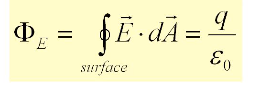The closed surface for which the flux is calculated is generally an imaginary or hypothetical surface, called a Gaussian surface.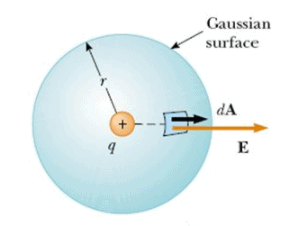Fig: Gaussian surface

Whenever we apply Gauss’s law we may choose a real or imaginary surface of any size and shape as our Gaussian surface. But selecting a proper size and shape for a Gaussian surface is a key factor for determining flux and electric field. Here are the list of different types of the Gaussian surfaces to be chosen for a given charge distribution.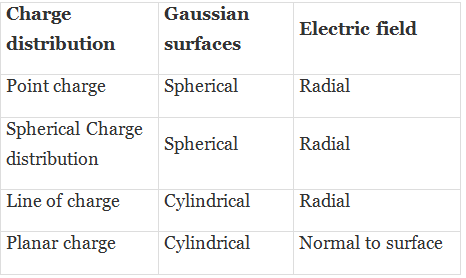Calculation of Electric Field using Gauss Law,
(a) Infinite sheet of uniform charge distribution (𝝈): We choose a small closed cylinder whose axis is perpendicular to plane as our gaussian surface. From symmetry we expect E to be directed perpendicular to the plane on both sides as shown, and to be uniform over the end caps of the cylinder.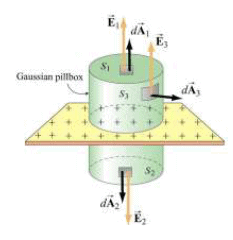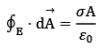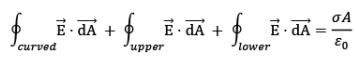by symmetry E is perpendicular to sheet. 𝚽 through lateral surface is zero as E is along x -axis and here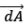is perpendicular to it.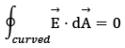Thus,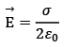The graph, given below, plots the values of Electric Field intensity across all possible positions from the sheet.

Note: That, the value of Electric field intensity is independent of the location where you are measuring it.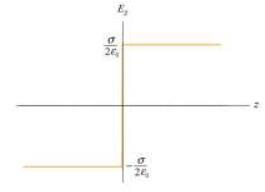We evaluated this electric field expression, when we calculated the electric field due to a non-conducting charged ring of radius R→∞.

(b) Uniformly charged non -conducting spherical shell: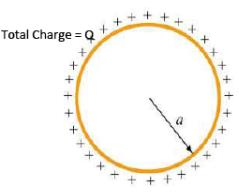Now, we would evaluate uniformly charged non-conducting spherical shell using Gauss Law given in the above figure. We would exploit spherical symmetry of the system here.

In order to calculate Electric Field we would divide the whole region into two types of spaces:

(i)Electric field inside the spherical shell
(ii)Electric Field outside the spherical shell

(i)Electric Field inside the spherical shell [r < a]:
We would imagine a spherical Gaussian surface of radius r concentric with the spherical shell (Radius a) such that r < a. Please refer to the figure given below.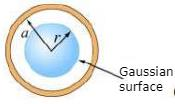Now, we apply Gauss Law for this Gaussian surface,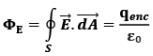we can observe, that r < a and the Gaussian surface encloses no net charge within itself. Thus, qenc = 0
Therefore,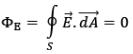Now, this integral can be zero only if either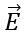= 0 oris perpendicular to the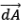at every point on the Gaussian surface. Due to situation symmetry, the latter is not possible. Hence, we conclude that,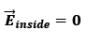Net electric Field inside the spherical shell is zero.

Note: Field cannot be along tangent of the gaussian surface. No tangential component can exist because of symmetry of charge distribution. Only direction possible is radial i.e. normal to the gaussian surface and hence,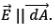(ii) Electric Field outside the spherical shell (r > a):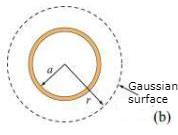We assume a closed spherical surface (gaussian surface) at the location where we intend to evaluate the Electric Field i.e. r distance from the centre (r > a)
By Gauss Law,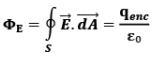Now, the Gaussian surface encloses the total charge on the spherical shell Q
Therefore, qenc = 𝑄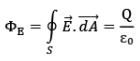Because of the spherical symmetry, the angle betweenand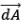at every point on the Gaussian surface is 0°.
Hence,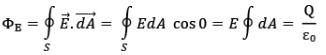∮ 𝑑𝐴 is read as the closed integral of the spherical Gaussian surface is the total surface area,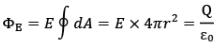Thus,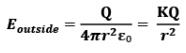Hence, Electric field outside the spherical shell at a distance r from the center of the shell is KQ/r2. It is interesting to note that this behaviour is similar to that of a point charge outside the shell.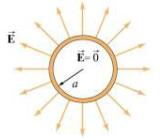The above diagram represents the electric field in the space due to the spherical non-conducting shell. We can also plot this behaviour on E vs r plot.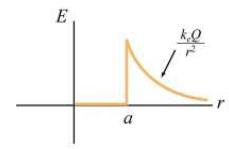Observe the hyperbolic decrease in electric field at r > a (outside of shell), similar to that of a point charge. While, the electric field inside the shell (r < a) remains zero.

(c) Uniformly charged non -conducting solid sphere: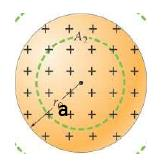Consider the above, non-conducting charged solid sphere of radius a having a total charge Q spread along its total volume.
We need to define the volumetric charge density for this sphere,
P = 𝑄/4π𝑎2
Again, in order to evaluate electric field intensity at all the points in a space, we will divide the space into two regions,
(i) Inside the sphere (r<a)
(ii) Outside the sphere (r>a)

(i) Inside the sphere (r<a): Now, consider an imaginary Gaussian surface of radius r such that (r<a), which would lie inside the solid sphere, as given in the following figure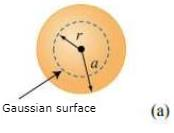For this Gaussian surface, we intend to apply the Gauss Law,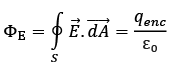Our aim is to evaluate the electric field intensity on any point on the Gaussian surface which is at a distance of r from the centre.
For the given Gaussian surface,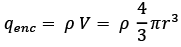Substituting it in Gauss Law,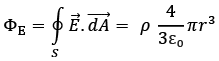Now, due to the spherical symmetry, at every point on the Gaussian surface, the Electric field vector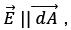Thus,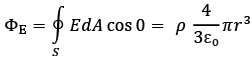Now, due to symmetry we all the points on the Gaussian surface will have same magnitude of Electric field E,
Therefore,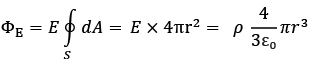Hence, 𝑬𝒊nside = Pr/3ε0 = KQr/a3

Note: That the Electric field at inside point of the solid sphere is directly proportional to r i.e. distance from the centre of the sphere. This is a linear increment.

(ii) Outside the solid sphere (r>a):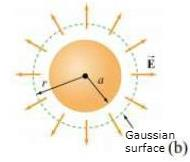Observe, that our gaussian surface will have a radius r which is now greater than a.
Again, by Gauss’ Law,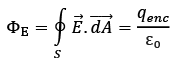Total charge enclosed by the Gaussian surface = Q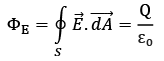Due the symmetry,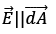Thus,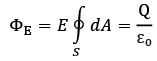Therefore,
E x 4πr2 = Q/ε0
Hence,
Eoutside = KQ/r2

Note: That even a uniformly charged solid sphere shows similar behaviour than that of a point charge, outside the sphere (r > a).

Field cannot be along tangent of the gaussian surface. No tangential component can exist because of symmetry of charge distribution. Only direction possible is radial i.e. normal to the gaussian surface and hence,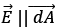SOLVED EXAMPLES

Example.1. There are three charges q1 = 3 C, q2 = 5 C and q3 = -2 C enclosed in a surface. What will be the total electric flux passing through the surface?
Solution. As according to Gauss’s Law, total electric flux equals 1/ ε0 times the total charge enclosed in a surface.
Total Charge = 3 C + 5 C – 2 C
Total Charge = + 6 C
Φ = q/𝜀0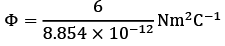Φ = 0.677 × 10−12Nm2C−1

Example.2. Consider three point charges –q, 2q, and 3q. The –q and 2q charge are enclosed in the surface, while the 3q charge is outside the surface S. What is the net electric flux? Also, comment about the electric field. Refer the image below.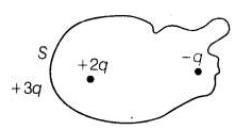Solution. According to Gauss’s law, we know that the net electric flux depends only on the net charge enclosed in the surface. Therefore, we would not consider the charge + 3q as it is outside our Gaussian surface.
Net Charge enclosed in the surface = 2q – q = q
By Gauss’ Law,
Φ = q/𝜀0
Important: Now, the electric field on any point in the space would depend on all three charges –q, +2q and 3q. Inside the surface, on the surface and outside the surface, everywhere the electric field depends on all the charges present.

Offer running on EduRev: Apply code STAYHOME200 to get INR 200 off on our premium plan EduRev Infinity!

,

,

,

,

,

,

,

,

,

,

,

,

,

,

,

,

,

,

,

,

,

;